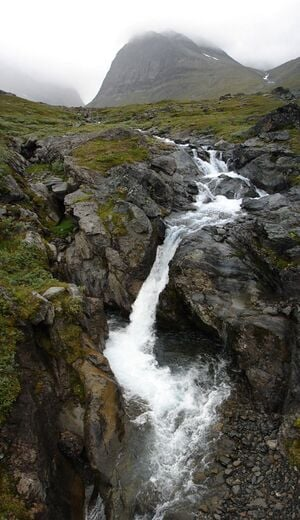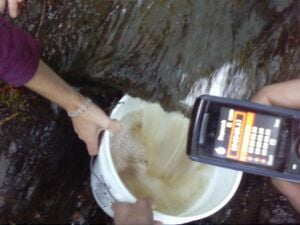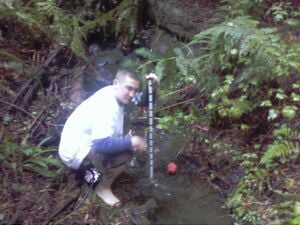Рисунок 1. Текущий горный ручей.

Расход — это общий объем жидкости, протекающей через фиксированную точку реки или ручья с течением времени. Это сравнимо со скоростью, с которой движется объем жидкости, как показано на рисунке 1. Объемный расход может быть измерен в различных единицах объема/времени, таких как:

• литров в секунду (л/с)
• Кубические футы в секунду (ft³/s)
• Галлоны в минуту (gal/min)
• Кубические метры в секунду (м³/с)

С помощью бытовых инструментов или специализированных счетчиков можно определить расход труб, канализационных систем и бытовых приборов. Люди используют данные о потоках для микрогидросистем , систем сточных вод , сбора дождевой воды, аудита воды , скорости оседания, статистики уровня грунтовых вод и другой информации, связанной с водой . Чтобы определить расход более крупных водоемов, таких как крупные реки или за плотинами, используются счетчики. 

На этой странице описаны несложные методы определения стока малых ручьев и рек, а также другие инструменты, которые можно использовать для этой цели.

## Метод 1: метод BucketРис. 2. Нахождение скорости потока с помощью ведра.

Метод ведра — это простой способ измерения скорости потока с помощью предметов домашнего обихода. Требуется секундомер, большое ведро и желательно два-три человека. Чтобы измерить скорость потока с помощью метода ведра:

1. Измерьте объем ведра или контейнера. Имейте в виду, что обычное ведро на 5 галлонов часто на самом деле меньше 5 галлонов.
2. Найдите место вдоль ручья, где есть водопад. Если ничего не удается найти, можно построить водопад с помощью плотины (см. рис. 4).
3. With a stopwatch, time how long it takes the waterfall to fill the bucket with water. Start the stopwatch simultaneously with the start of the bucket being filled and stop the stopwatch when the bucket fills. The bucket should not be filled by holding it below the surface of the stream because it is not the true flow rate.
4. Record the time it takes to fill the bucket.
5. Repeat steps two and three about six or seven times and take the average. It is a good idea to do a few trial runs before recording any data so that one can get a feel for the timing and measurements required.
6. Only eliminate data if major problems arise such as debris from the stream interfering with the flow.
7. The flow rate is the volume of the bucket divided by the average time it took to fill the bucket.
Bucket method data for flow (example)
Trial NumberTime (seconds)Bucket Volume (gallons)
113.25
2145
314.55
4135
513.45
613.15

Here is an example using data found for the flow rate of the Jolly Giant Creek on Cal Poly Humboldt grounds: Using this data, the volumetric flow rate (Q) is equal to the volume of the bucket (V) divided by the average time (t).

Q=v/t{\displaystyle Q=v/t}where t=13.2s+14s+14.5s+13s+13.4s+13.1s6trials=13.5seconds{\displaystyle t={\frac {13.2s+14s+14.5s+13s+13.4s+13.1s}{6trials}}=13.5seconds}so t=13.5seconds{\displaystyle t=13.5seconds}and V=5gallons{\displaystyle V=5gallons}Q=Vt=5gallons13.5seconds=0.37gallonssecond{\displaystyle Q={\frac {V}{t}}={\frac {5gallons}{13.5seconds}}=0.37{\frac {gallons}{second}}}So the flow rate is 0.37 gallons/second or Q = 0.37 gal/sec * 60 sec/min = 22.2 gallons/minute.

Therefore the flowrate (Q) is 22.2 GPM.

## Method 2: Float methodFigure 3. Finding the flow rate using a float and a meter stick.

The float method (also known as the cross-sectional method) is used to measure the flow rate for larger streams and rivers. It is found by multiplying a cross sectional area of the stream by the velocity of the water. To measure the flow rate using the float method:

1. Locate a spot in the stream that will act as the cross section of the stream.
2. Using a meter stick, or some other means of measurement, measure the depth of the stream at equal intervals along the width of the stream (see Figure 3). This method is similar to hand calculating a Riemann sum for the width of the river.
3. Once this data is gathered, multiply each depth by the interval it was taken in and add all the amounts together. This calculation is the area of a cross section of the stream.
4. Decide on a length of the stream, typically longer than the width of the river, to send a floating object down (oranges work great).
5. Using a stopwatch, measure the time it takes the float to travel down the length of stream from step 4.
6. Repeat step five 5-10 times and determine the average time taken for the float to travel the stream. Throw the float into the water at different distances from the shoreline in order to gain a more accurate average.
7. Divide the stream length found in step 4 by the average time in step 6 to determine the average velocity of the stream.
8. The velocity found in step 7 must be multiplied by a friction correction factor. Since the top of a stream flows faster than the bottom due to friction against the stream bed, the friction correction factor evens out the flow. For rough or rocky bottoms, multiply the velocity by 0.85. For smooth, muddy, sandy, or smooth bedrock conditions, multiply the velocity by a correction factor of 0.9.
9. The corrected velocity multiplied by the cross sectional area yields the flow rate in volume/time. (Be sure to keep consistent units of length/distance when measuring the cross section and the velocity e.g. meters, feet)

## Method 3: Weirs

Weirs are small dams that can be used in measuring flow rate for small to medium sized streams (a few meters or wider). They allow overflow of the stream to pour over the top of the weir, creating a waterfall, as seen in Figure 4. Weirs increase the change in elevation making the streamflow more consistent which makes flow rate measurements more precise. However, it is very important that all the water in the stream be directed into the weir for it to accurately represent the stream flow. It is also important to keep sediment from building up behind the weir. Sharp crested weirs work best. There are many different types of weirs which include broad crested weirs, sharp crested weirs, combination weirs, V-notch weirs and minimum energy loss weirs.

## Method 4: Meters

Meters are devices that measure the stream flow by directly measuring the current. There are many different types of meters but the most common are the Pygmy meter, the vortex meter, the flow probe, and the current meter, described below: